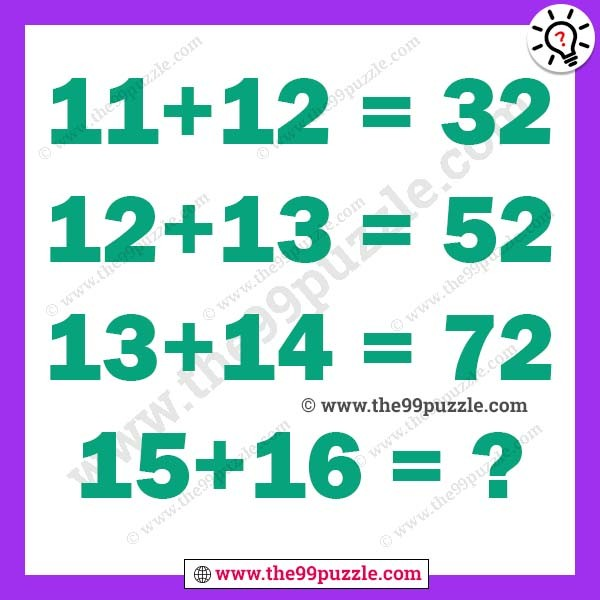# Easy math puzzles with answer – Puzz154

Can you solve these easy math puzzles? This puzzle is very easy and simply apply a trick. If you observe carefully you can understand the math puzzle logic and calculate the last equation.

11+12=32

12+13=52

13+14=72

15+16=?###### Explanation:

11+12=23(Reverse the number)=32

12+13=25(Reverse the number)=52

13+14=27(Reverse the number)=72

15+16=31(Reverse the number)=13